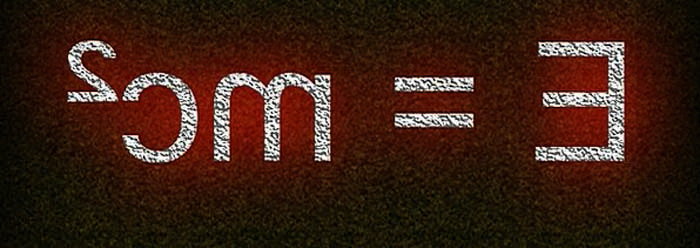Medical Entrance Exams

PAHUNT Physics Detailed SyllabusUnit and Dimensions, Dimensional Analysis, S.I, Units, Motion in two dimensions cases of uniform velocity and uniform acceleration, general relation among position and velocity, uniform circular motion and inertia. Newton’s laws of motion, Conservation of momentum and energy.

Static and kinetic friction, work energy and power elastic collisions, potential energy, gravitational potential energy and its angular conservations to its kinetic energy, Potential energy of a spring. Rigid body rotation and conservation of its momentum, moment of inertia, theorems of parallel and perpendicular axis. ( moment of inertia of uniform ring, disc, thin rod and cylinder only).

Acceleration due to gravity and its variation, universal law of gravitation, geostationary satellites, escape velocity.

Hooke’s law, young’s modulus, shear and bulk modulus, surface energy and surface tension, kinetic theory of gases, gas laws, kinetic energy and temperature.

Specific heats and constant volume and constant pressure mechanical equivalent of heat, isothermal and adiabatic processes. Heat conduction in one dimension, convection and radiation, Stefan’s law and Newton’s law of cooling.

Periodic motion, simple harmonic motion, Oscillations due to spring. Wave motion, principle of superposition, progressive and stationery waves, beats and Doppler effect.

Wave nature of light, interference , young’s double slit experiment, velocity of light and Doppler effect in light.

Reflection, refraction, total internal reflection, curved mirrors, lenses, mirror and lens formulae. Dispersion in prism, absorption and emission spectra. The human eye, defects of vision, magnification and resolving power of telescope and microscope.

“e” and “e/m” for and electron, Einstein’s photoelectric equation, photocells. Bohr model of the atom, Hydrogen spectrum, composition of nucleus, atomic masses and isotopes, radioactivity, laws of radio active decay, decay constant, half life and mean life, mass energy relation, fissions, X- ray, properties and uses.

Elementary ideas of conductor, semi conductor and insulator, intrinsic and extrinsic semiconductors, p-n junction as a rectifier. Bar magnet, lines of force, torque on a bar magnet due to magnetic field, earth’s magnetic field , tangent galvanometer, vibration maganetometer.

Coulomb’s law of electrostatic, dielectric constant, electric field and potential due to a point charge, dipole, dipole field, Guass’s law in a simplegeometrics.

Electrostatic potential, capacitance, parallel plate and spherical capacities in series and parallel, energy of a capacitor.Electric current, Ohm’s law, Kirchhoffs laws, resistances in series and parallel temperature dependence of resistance, Wheat stone bridge, potentiometer.Measurement of voltage as currents. Electric power, heating effects of currents, chemical effects and law of electrolysis thermoelectricity Blot Savart law, magnetic fields due to a straight wire circular loop and solenoid. Force on a moving charge in a magnetic field(Lorentz force), magnetic moment of a current loop, effect of a uniform magnetic field of a current loop, forces between two currents, moving coil, galvonometer, ammeter and voltmeter.

Electromagnetic induction induced e.m.f., Faradays law, Lenz’s law, self and mutual inductance alternating currents, impedance and reactance, growth and decay of current in L-R circuit, elementrary idea if dynamo and transformer.